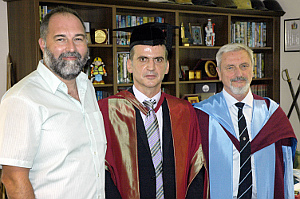EM Waves and the Human EyeHrvoje Dodig graduated from the University of Split, Croatia, and obtained his MPhil degree at the Wessex Institute of Technology (WIT). Hrvoje continued his research at WIT and has successfully passed his PhD viva with the thesis “EM Waves and the Human Eye”. His external examiner was Dr. Stuart Porter from the University of York and the internal examiner was Dr. Victor Popov.

The objective of the research was to find a feasible computational method for 3D computation of time-harmonic electromagnetic fields inside the biological tissue induced by external RF excitation. The calculated field was used as an input to the bio-heat equation in order to compute thermal elevation caused by an external field. The computational model was then applied to the human eye.

In order to account for inhomogeneity of the human tissues the coupled BEM-FEM approach was implemented. Because current EM theory requires that tangential components of EM fields across regions with varying material properties are preserved, volume and surface edge elements were used.

Stratton-Chu equations are commonly used in the field of electromagnetics as a boundary integral equation to represent fields caused by charges and currents on the surface of the problem. However, in the thesis these equations were generalized and it was shown that whenever the vector field satisfies the vector Helmholtz equation, Stratton-Chu equations are applicable.

When the Stratton-Chu integral equation is used with BEM, one has to deal with surface hypersingular integrals. Because such integrals cannot be solved analytically, they are calculated numerically which is in this case an extremely time consuming problem. This was circumvented in the thesis by recasting these surface integrals to contour integrals around the edge of the element. The advantage of this approach is to reduce computation time; as it offers good possibilities for applying a simple adaptive algorithm and in fact any adaptive integral calculation could be used. However, in the thesis, a new adaptive strategy was developed which is considerably faster than present methods and it was compared with the Gauss-Patterson computational scheme. Furthermore, it was shown that the method of recasting to contour integral works not only for the Helmholtz equation; it is possible to recast other integral equations to contour integrals as well. This recasting to contour integrals would not be possible without the mathematical apparatus developed in the thesis. One of the more interesting points of this mathematical apparatus is the development of new stokes-like theorem and a new “gradient” theorem for vector fields.

One important parameter used to characterise the validity of the numerical method for the EM scattering problem is the calculation of the radar cross section (RCS). Because it was not clear from the literature how to calculate RCS only from coefficients associated with edge elements, the method for RCS computation from these coefficients was developed. The results of RCS computation follow closely known examples in the literature. Furthermore, it is established in the literature that electric field integral formulation (EFIE) produces significant errors at certain frequencies for the example of a coated sphere. Thus, in the thesis, EFIE was deliberately used to compute RCS at frequencies at which the computational method was bound to fail. However, the discrepancy between numerical and theoretical results was not observed. It was concluded that the most likely reason for this lies in the method used to compute boundary integrals, as the error was controlled using adaptive methods. The numerical method was compared with the Mie series solution as well and no significant errors were observed.

Finally, the numerical method was used to compute the thermal rise in the eye for plane wave excitation with the power of 10 mW/m2. The computed SAR was well within the safety margins prescribed by ICNIRP, and once again it was shown that the plane wave with power density of 10 mW/mis not harmful to humans, in terms of thermal rise, in the frequency range from 1 GHz to 6 GHz.